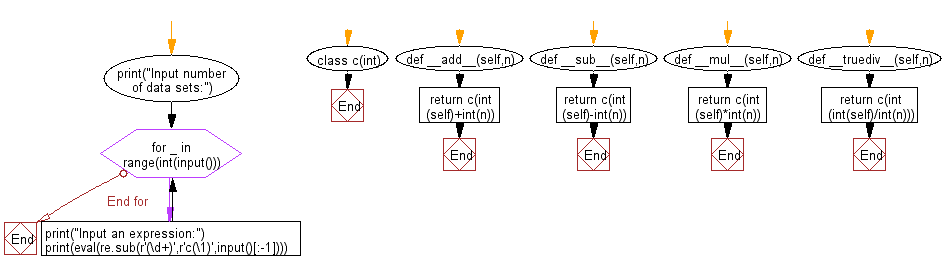﻿ Python: Reads a given expression and evaluates it - w3resource# Python: Reads a given expression and evaluates it

## Python Regular Expression: Exercise-52 with Solution

Write a Python program that reads a given expression and evaluates it.

Terms and conditions:
The expression consists of numerical values, operators and parentheses, and the ends with '='.
The operators includes +, -, *, / where, represents, addition, subtraction, multiplication and division.
When two operators have the same precedence, they are applied to left to right.
You may assume that there is no division by zero.
All calculation is performed as integers, and after the decimal point should be truncated Length of the expression will not exceed 100.
-1 × 10 9 ≤ intermediate results of computation ≤ 10 9

Sample Solution:-

Python Code:

``````#https://bit.ly/2lxQysi
import re
print("Input number of data sets:")
class c(int):
return c(int(self)+int(n))
def __sub__(self,n):
return c(int(self)-int(n))
def __mul__(self,n):
return c(int(self)*int(n))
def __truediv__(self,n):
return c(int(int(self)/int(n)))

for _ in range(int(input())):
print("Input an expression:")
print(eval(re.sub(r'(\d+)',r'c(\1)',input()[:-1])))
```
```

Sample Output:

```Input number of data sets:
2
Input an expression:
4-2*3=
-2
Input an expression:
4*(8+4+3)=
60
```

Flowchart:Python Code Editor:

Have another way to solve this solution? Contribute your code (and comments) through Disqus.

What is the difficulty level of this exercise?

Test your Python skills with w3resource's quiz

﻿

## Python: Tips of the Day

Getting the last element of a list:

some_list[-1] is the shortest and most Pythonic.

In fact, you can do much more with this syntax. The some_list[-n] syntax gets the nth-to-last element. So some_list[-1] gets the last element, some_list[-2] gets the second to last, etc, all the way down to some_list[-len(some_list)], which gives you the first element.

You can also set list elements in this way. For instance:

```>>> some_list = [1, 2, 3]
>>> some_list[-1] = 5 # Set the last element
>>> some_list[-2] = 3 # Set the second to last element
>>> some_list
[1, 3, 5]
```

Note that getting a list item by index will raise an IndexError if the expected item doesn't exist. This means that some_list[-1] will raise an exception if some_list is empty, because an empty list can't have a last element.

Ref: https://bit.ly/3d8TfFP